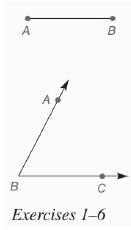Chapter 7.CR, Problem 4CR### Elementary Geometry for College St...

6th Edition
Daniel C. Alexander + 1 other
ISBN: 9781285195698

#### Solutions

Chapter
Section### Elementary Geometry for College St...

6th Edition
Daniel C. Alexander + 1 other
ISBN: 9781285195698
Textbook Problem
1 views

# In Review Exercises 1 to 6, use the figure shown.Construct an isosceles triangle with vertex angle B and an altitude with the length of A B ¯ from vertex B to the base.To determine

To construct: An isosceles triangle with vertex angle B and an altitude with the length of AB¯

from vertex B to the base.

Explanation

Given:

Construct an isosceles triangle with vertex angle B and an altitude with the length of AB¯

from vertex B to the base.

Calculation:

Consider the altitute of the isosceles triangle as AB¯

### Still sussing out bartleby?

Check out a sample textbook solution.

See a sample solution

#### The Solution to Your Study Problems

Bartleby provides explanations to thousands of textbook problems written by our experts, many with advanced degrees!

Get Started

#### Find more solutions based on key concepts